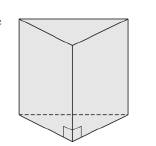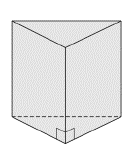Chapter 9.1, Problem 16E### Elementary Geometry for College St...

6th Edition
Daniel C. Alexander + 1 other
ISBN: 9781285195698

#### Solutions

Chapter
Section### Elementary Geometry for College St...

6th Edition
Daniel C. Alexander + 1 other
ISBN: 9781285195698
Textbook Problem
5 views

# For the right triangular prism, suppose that the sides of the triangular base measure 4 m, 5 m, and 6 m. The altitude is 7 m.a) Find the lateral area of the prism.b) Find the total area of the prism.c) Find the volume of the prism.To determine

a)

The lateral area of the prism.

In the accompanying right triangular prism, each base edge measures 4 m, 5 m, and 6 m.

The altitude is 7 m.Explanation

The lateral area of any solid is the area of all the number of lateral sides that it have, excluding the area of the top and the bottom of the solid.

The lateral area (L) of any solid prison with the perimeter of the base of the prism as (P) and altitude ‘h’ is given by the formula

L=Ph.

Here, P is the perimeter of any one of the base of the prism and which is given by the sum of all the sides of the base.

Calculation:

Given,

The measure of the base edges of the triangular prism =4 m, 5 m, and 6 m.

The altitude of the prism =7m.

The lateral area of any prism of altitude ‘h’ and of base perimeter P is given by

L=hP

Here, we have

h=7 m.

First, let us find the perimeter of the base of the prism

To determine

b)

The total area of the prism.

In the accompanying right triangular prism, each base edge measures 4 m, 5 m, and 6 m.

The altitude is 7 m.To determine

c)

The volume of the prism.

In the accompanying right triangular prism, each base edge measures 4 m, 5 m, and 6 m.

The altitude is 7 m.### Still sussing out bartleby?

Check out a sample textbook solution.

See a sample solution

#### The Solution to Your Study Problems

Bartleby provides explanations to thousands of textbook problems written by our experts, many with advanced degrees!

Get Started

#### Sketch the graphs of the equations in Exercises 512. xy=4

Finite Mathematics and Applied Calculus (MindTap Course List)

#### Find dy/dx by implicit differentiation. 14. ey sin x = x + xy

Single Variable Calculus: Early Transcendentals, Volume I

#### Find the inverse of the matrix .

Mathematical Applications for the Management, Life, and Social Sciences

#### What is the value of (X + 1) for the following scores: 0, 1, 4, 2? a. 8 b. 9 c. 11 d. 16

Essentials of Statistics for The Behavioral Sciences (MindTap Course List)

#### Evaluating Limits Use a graphing utility to evaluate limx0sinnxx

Calculus: Early Transcendental Functions (MindTap Course List)

#### j × (−k) = i −i j + k −j − k

Study Guide for Stewart's Multivariable Calculus, 8th

#### Define content analysis and archival research.

Research Methods for the Behavioral Sciences (MindTap Course List)